# UGC NET COMMERCE SOLVED PAPERS 2017-19 - UGC NET COMMERCE December 2018

>>>>>>>>UGC NET COMMERCE December 2018

• A

(i) and (iv)• B

(ii) and (iii)• C

(ii), (iii) and (iv)• D

(i), (ii) and (iii)• A

Improved data integrity• B

Centralized control• C

High acquisition costs• D

Shared data• A

1• B

2• C

3• D

4• Option : D
• Explanation : Probable Error and Standard Error of Coefficient of Correlation
The probable error (PE) of coefficient of correlation indicates extent to which its value depends on the condition of random sampling. If r is the calculated value of correlation coefficient in a sample of n pairs of observations, then the standard error SEr of the correlation coefficient r is given by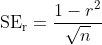The probable error of the coefficient of correlation is calculated by the expression: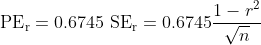Thus with the help of PEr we can determine the range within which population coefficient of correlation is expected to fall using following formula: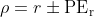where ρ (rho) represents population coefficient of correlation.
Remarks
1. If r < PEr then the value of r is not significant, that is, there is no relationship between two variables of interest.
2. If r > 6PEr then value of r is significant, that is, there exists a relationship between two variables.
Illustration: If r = 0.8 and n = 25, then PEr is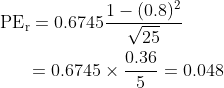Thus the limits within which population correlation coefficient (ρr) should fall are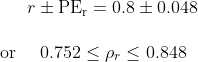• A

Strategic control• B

Annual-plan control• C

Efficiency control• D

Profitability control• Option : A
• Explanation :